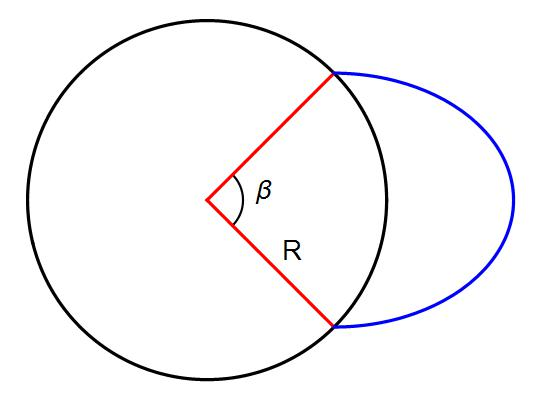# Project GravitaA particle is projected from the surface of the earth (of radius R and mass M) with speed $V$ given by

$V=\sqrt{\frac{GM}{R}}$ at a certain angle from the local horizantal as shown in the figure such that the angle subtended by arc between launching and landing site at earth's centre is $\beta=\frac{\pi}{2}$

Maximum separation of the particle from the centre of the earth is xR. find $\lfloor 10x\rfloor$

Consider the earth to be uniformly dense and air resistance to be absent.

×

Problem Loading...

Note Loading...

Set Loading...